Magnetic fields are almost everywhere: automotive drives, power supply or magnetic resonance imaging. Without magnetic fields the world wouldn't be like it is today. In this section we will explain and examine the magnetic flux density, coils, elctromagnets and magnetic force.

1 The effect of an bar magnet on a welding arc

A bar magnet is approaching to a welding arc. As a result of this the welding arc is distracted. Watch yourself in the short film.

Magnetic forces always occur pairwise. So beside the bar magnet there must be a second magnetic field. That is the point. Where is it?

By the way: There is an attraction between the N-pole and the S-pole. But same poles do repell each other. The earth acts as if there is a huge bar magnet inside it. The N-pole of a bar magnet will be attracted toward the northern hemisphere. So there must be a imaginary S-pole far north.

Are you interessted? Here you will find more answers ...

2 Magnetic field pattern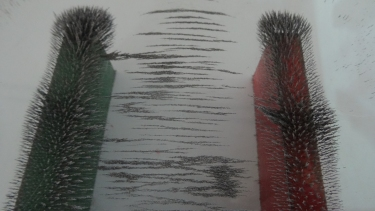Even some people state to be sensitive to a magnetic field, humans do not have any sensors for the magnetic field. But we are able to recognize the forces of a magnetic field: a compass or a magnet holding something on the steel dor of the fridge.

The magnetic field pattern can made be visible with iron fillings on top of a horse-shoe magnet. In between is a homogeneous field, outside we are talking of inhomogenous field. The field lines do have a direction since the iron fillings will move as you start to move the magnet.

The stronger the magnetic field, the more densely packed the lines of flux. So we describe the strength of the magnetic field by the magnetic flux density B, which is measured in Tesla (T).

3 The magnetic effect of a current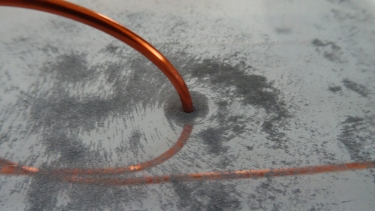Around a long straight wire with a current flow inside we observe a circular magnetic field. However to observe this there is current of at least 20 A necessary.

If you use magnet neadels instead of iron fillings, you will notice a change in direction if the direcetion of current changes. This leads to the right-hand grip rule:

If your right thumb points in the direction of the current, your fingers then curl in the direction of the lines of flux.

Around a wire the magnetic flux density decreases with the distance $r$: $B=\frac{\mu_0 I}{2\pi r}$, in which the constant $\mu_0=4\pi \cdot 10^{-7}~TmA^{-1}$ is the permeability of free space.

4 magnetic field pattern and strength of a solenoidIf you wrap a long wire we are talking of a coil. A solenoid is a long coil with a large number of turns of wire. The result of the superposition of each magnetic field is a homogeneous field pattern inside the solenoid. The pattern is identical to the field of a bar magnet. The difference: We can switch it on/off and adjust the strength of the magnetic field.

The stronger the current $I$ and the more turns of wire $n$ the stronger the magnetic flux density. If a solenoid is in a vacuum or air we can write:

$$B=\mu_0 \cdot n \cdot I$$
5 Magnetic materials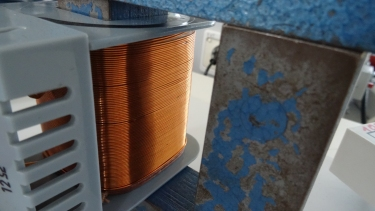Electrons spin in each atom. So each electron acts like a tiny electric current and produces a tiny magnetic field. In some materials the magnetic effects of all electrons cancel in other they line up. In ferromagnetic materials like iron, cobalt and nickel the tine magnetic fields line up to a strong magnetic field.

So a ferromagnetic core in a solenoid increases the magnetic strength. With the permeability of materials $µ_r$ which is up to 10,000 for iron, 1 in vacuum and less then 1 for copper we can write:

$$B=\mu_0 \cdot \mu_r \cdot n \cdot I$$
6 Magnetic force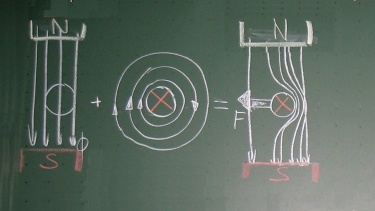A wire carrying current placed in a magnetic field feels a force. The two magnetic fields interact with each other. If the direction of the two patterns are opposite the fields are destructive. If the direction is identical they line up constructive to a strong field resulting in a force on the wire. We can construct the direction of force with the right hand rule and the knowledge that densly packed field lines result in a pushing force.

The stronger the magnetic flux density $B$, the higher the current $I$, the larger the length $l$ of the conductor in the field and the more vertical the angle $\theta$ between the magnetic field and the conductor the greater the force $F$: $$F=B\cdot I\cdot l\cdot sin~\theta$$

7 Magnetic force on a moving charge

Charged particles from the outer space get trapped by the magnetic field of earth and produce the spectacular glow in the sky shown in the video. The force (Lorentz) on a moving charge with its charge $Q$, velocity $v$ and angle $\theta$ can be written to: $$F=B\cdot Q \cdot v \cdot sin~\theta$$

ideas of
R. Brugger, FTA15 Elektronikschule Tettnang
K. Johnson et al., "Advanced Physics for You"

#### Question 1 construction of magnetic field pattern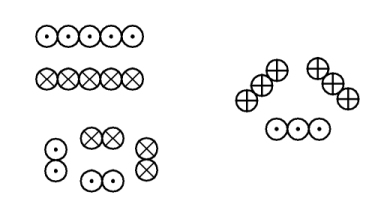Construct the magnetic field pattern around the current carrying wires#### Question 2 magnetic force

Which description fits?1. Around a current carrying wire, a magnetic field is ...

2. The direction of the magnetic field ...1. The magnetic field between two poles is directed from ...

2. opposite poles ...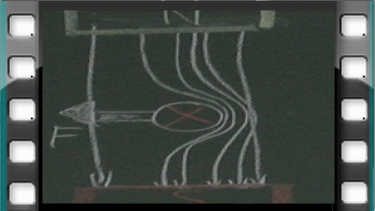1. On the right side of the wire, both magnetic fields interact ...

2. The direction of force is towards the ...

#### Experiment 1 make magnetic field pattern visible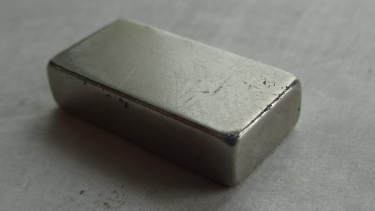All you need is a bar magnet, acrylic glass and iron fillings.

Before you put the iron fillings on the bar magnet put a acrylic glass in between.

Hint: make a video of the experiment.

• How does the magnetic field pattern look like?

• Where is the field homogeneous and where inhomogenous?

• Does the direction change if we flip the manget?

• Add a second bar magnet and repeat the experiement.

• magnetic field pattern of a horse shoe magnet:### First relax ...

Here you can find the world's simplest electric train.
Can you explain how it works?

#### Question 3 flux density

Calculate the magnetic flux density1. Calculate the magnetic flux density at a distance of 2.5 cm from a long straight wire carrying a current of 2.0 A.
2. Close to a wire carrying a current of 4.0 A the magnetic flux density is $4.0\cdot 10^{-5}$ T. How far is the distance from the wire?
3. A solenoid of length 12 cm has 4800 turns per meter, the current through it is 2.5 A. Calculate the magnetic flux densty at the centre of the solenoid.
1. magnetic flux density:
$B=\frac{\mu_0I}{2\pi r}=1.6 \cdot 10^{-5}~T$

2. distance from the wire:
$r=\frac{\mu_0I}{2\pi B}=2~cm$

3. magnetic flux density:
$B=\mu_0 n I = \mu_0 \frac{4800}{0.12} I = 0.126~T$

#### Question 4 construction of magnetic force

Construct the resulting magnetic field and force

1. magnetic field (blue) to the right, current (red) into the board2. magnetic field (blue) and current (red) to the right1. magnetic field (blue) into the board, current (red) to the right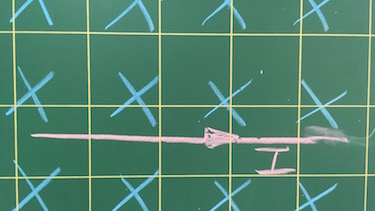2. magnetic field (blue), current (red) upwardsResuslting magnetic field and force: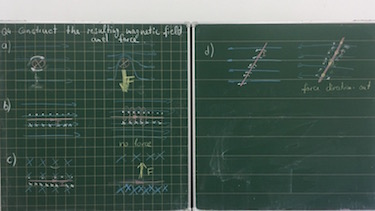#### Question 5 The magnetic force on a moving charge

An electron with a charge of $Q=e=-1.6 \cdot 10^{-19}~As$ is moving with $v=5 \cdot 10^{7}~m/s$ perpendicular to an uniform magnetic field with flux density $B=6.25 \cdot 10^{-2}~T$. The mass of the electron is $m=9.1 \cdot 10^{-31}~kg$.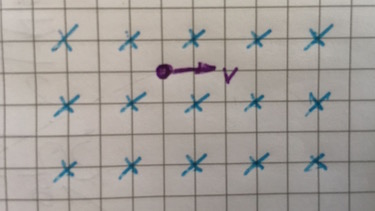1. Construct the direction of the force.
2. Calculate the force.
4. Calculate the time period T.
5. Explain how mass spectrometers are used to identify different isotopes in a sample of material.
1. Resuslting magnetic field and force: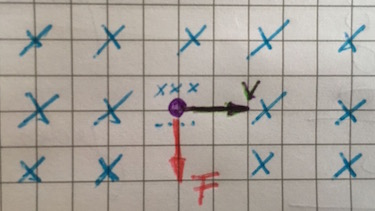2. magnetic force:
$F=BQv= -5 \cdot 10^{-13}~N$

$r=\frac{mv}{BQ}= 4.55 \cdot 10^{-3}~m$
$T=\frac{2\pi m}{BQ}= 5.72 \cdot 10^{-10}~s$